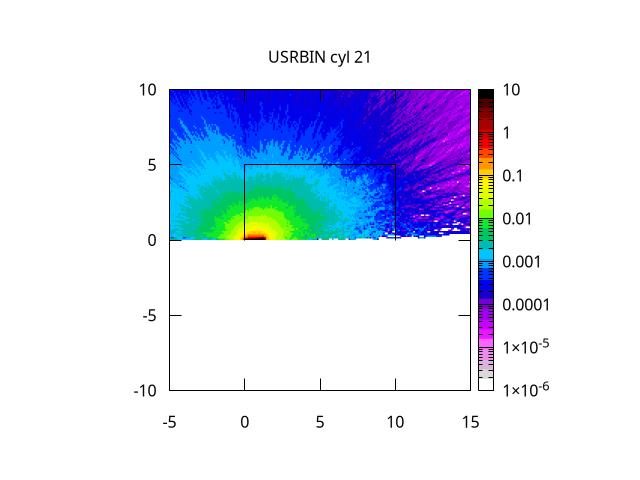# Can the total figure be plot in flair?

In a simulation using fluka, the EM energy was calculated in a cylinder volume,
the result was plotted in flair, here is the figure:

y axis represents the radius direction, only half figure was plotted,
but i want the total figure, it can be achieved in flair?

here is the .inp file:
MeshTally.inp (1.9 KB)

Dear @Newconcept_1979,

You have defined a cylindrical R-Phi-Z scoring. A cylinder with a negative radius makes no physical sense, R is defined as positive quantity, therefore R=0 is the minimum value R can achieve.

Dear @Newconcept_1979,

in addition to @amario’s answer, if you plot the results using the Geometry editor / viewer, you can visualize both sides of the cylinder.

See:

vs.Cheers,
David